JEE  >  Competition Level Test: Definite And Indefinite Integral

# Competition Level Test: Definite And Indefinite Integral

Test Description

## 30 Questions MCQ Test Mathematics (Maths) Class 12 | Competition Level Test: Definite And Indefinite Integral

Competition Level Test: Definite And Indefinite Integral for JEE 2023 is part of Mathematics (Maths) Class 12 preparation. The Competition Level Test: Definite And Indefinite Integral questions and answers have been prepared according to the JEE exam syllabus.The Competition Level Test: Definite And Indefinite Integral MCQs are made for JEE 2023 Exam. Find important definitions, questions, notes, meanings, examples, exercises, MCQs and online tests for Competition Level Test: Definite And Indefinite Integral below.
Solutions of Competition Level Test: Definite And Indefinite Integral questions in English are available as part of our Mathematics (Maths) Class 12 for JEE & Competition Level Test: Definite And Indefinite Integral solutions in Hindi for Mathematics (Maths) Class 12 course. Download more important topics, notes, lectures and mock test series for JEE Exam by signing up for free. Attempt Competition Level Test: Definite And Indefinite Integral | 30 questions in 60 minutes | Mock test for JEE preparation | Free important questions MCQ to study Mathematics (Maths) Class 12 for JEE Exam | Download free PDF with solutions
 1 Crore+ students have signed up on EduRev. Have you?
Competition Level Test: Definite And Indefinite Integral - Question 1

### Let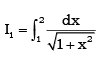and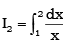then

Detailed Solution for Competition Level Test: Definite And Indefinite Integral - Question 1

We have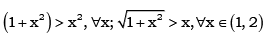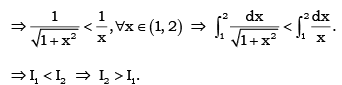Competition Level Test: Definite And Indefinite Integral - Question 2

###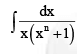is equal to

Detailed Solution for Competition Level Test: Definite And Indefinite Integral - Question 2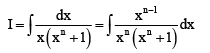Putting xn = t so that n xn–1 dx = dt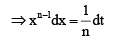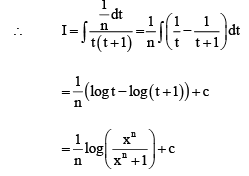Competition Level Test: Definite And Indefinite Integral - Question 3

###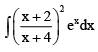is equal to

Detailed Solution for Competition Level Test: Definite And Indefinite Integral - Question 3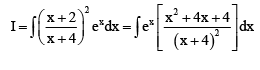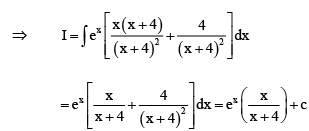Competition Level Test: Definite And Indefinite Integral - Question 4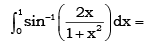Detailed Solution for Competition Level Test: Definite And Indefinite Integral - Question 4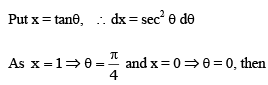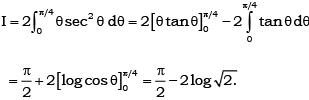Competition Level Test: Definite And Indefinite Integral - Question 5

If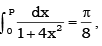then P =

Detailed Solution for Competition Level Test: Definite And Indefinite Integral - Question 5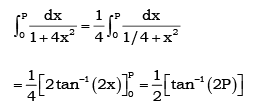Comparing it with the given value, we get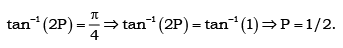Competition Level Test: Definite And Indefinite Integral - Question 6

The value of integral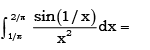Detailed Solution for Competition Level Test: Definite And Indefinite Integral - Question 6

put t = 1/x ⇒ dt = -1/x2 as t = π/2 and π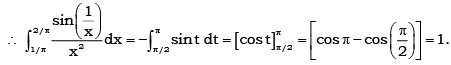Competition Level Test: Definite And Indefinite Integral - Question 7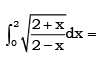Detailed Solution for Competition Level Test: Definite And Indefinite Integral - Question 7

Put x = 2 cos θ ⇒ dx = - 2 sin θ dθ, then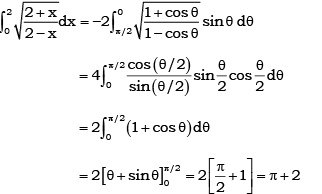Competition Level Test: Definite And Indefinite Integral - Question 8

If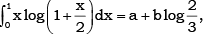then

Detailed Solution for Competition Level Test: Definite And Indefinite Integral - Question 8

Integrate it by parts taking  log (1+ x/2 )as first function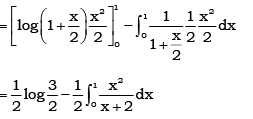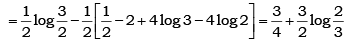Competition Level Test: Definite And Indefinite Integral - Question 9

The value of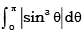is

Detailed Solution for Competition Level Test: Definite And Indefinite Integral - Question 9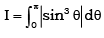Since sinq is positive in interval (0, π)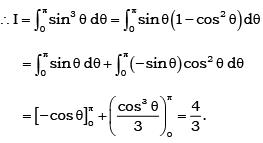Competition Level Test: Definite And Indefinite Integral - Question 10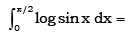Detailed Solution for Competition Level Test: Definite And Indefinite Integral - Question 10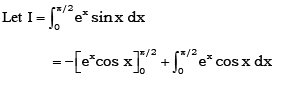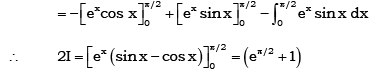Competition Level Test: Definite And Indefinite Integral - Question 11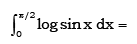Detailed Solution for Competition Level Test: Definite And Indefinite Integral - Question 11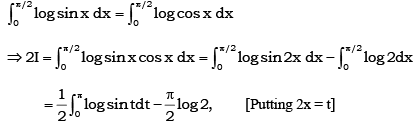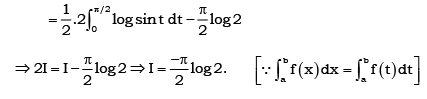Competition Level Test: Definite And Indefinite Integral - Question 12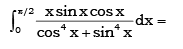Detailed Solution for Competition Level Test: Definite And Indefinite Integral - Question 12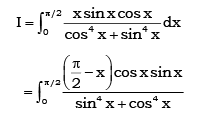By adding (i) and (ii), we get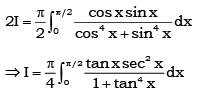Now, Put tan2x = t, we get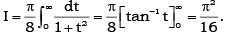Competition Level Test: Definite And Indefinite Integral - Question 13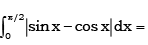Detailed Solution for Competition Level Test: Definite And Indefinite Integral - Question 13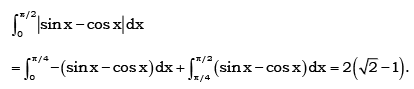Competition Level Test: Definite And Indefinite Integral - Question 14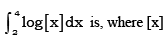denotes the greater integer less than or equal to x

Detailed Solution for Competition Level Test: Definite And Indefinite Integral - Question 14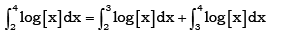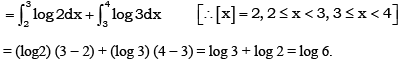Competition Level Test: Definite And Indefinite Integral - Question 15

If [x] denotes the greater integer less than or equal to x, then the value of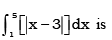Detailed Solution for Competition Level Test: Definite And Indefinite Integral - Question 15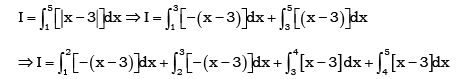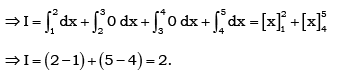Competition Level Test: Definite And Indefinite Integral - Question 16

If f(x) = tan x - tan3 x + tan5 x - …… to ∞ with 0 < x < π/4, then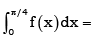Detailed Solution for Competition Level Test: Definite And Indefinite Integral - Question 16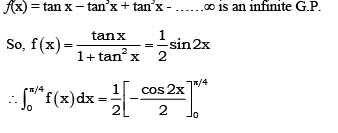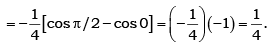Competition Level Test: Definite And Indefinite Integral - Question 17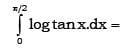Detailed Solution for Competition Level Test: Definite And Indefinite Integral - Question 17

I = ∫0 π2 log(tan x).dx
I = ∫0 π2 log(cot x).dx
Adding both the equations, we get
2I = ∫0 π2 log(tanx) + log(cot x) dx
2I = ∫0 π2 log(1).dx
= 0

Competition Level Test: Definite And Indefinite Integral - Question 18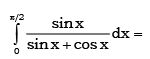Detailed Solution for Competition Level Test: Definite And Indefinite Integral - Question 18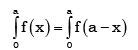Competition Level Test: Definite And Indefinite Integral - Question 19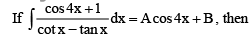Detailed Solution for Competition Level Test: Definite And Indefinite Integral - Question 19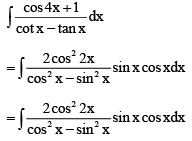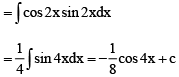Competition Level Test: Definite And Indefinite Integral - Question 20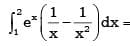Detailed Solution for Competition Level Test: Definite And Indefinite Integral - Question 20

f’(x) = -1/x2
Thus, ∫(1 to 2)ex(1/x - 1/x2)dx
= [ex/x](1 to 2) + c
= e2/2 - e

Competition Level Test: Definite And Indefinite Integral - Question 21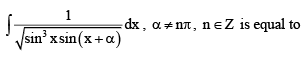Detailed Solution for Competition Level Test: Definite And Indefinite Integral - Question 21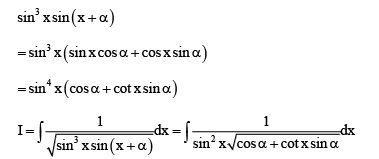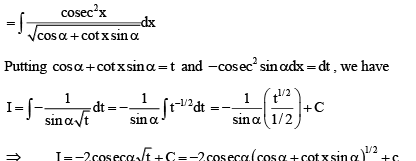Competition Level Test: Definite And Indefinite Integral - Question 22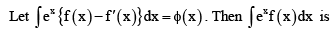Detailed Solution for Competition Level Test: Definite And Indefinite Integral - Question 22

Here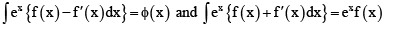on adding we get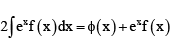Competition Level Test: Definite And Indefinite Integral - Question 23

If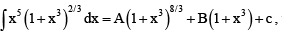then

Detailed Solution for Competition Level Test: Definite And Indefinite Integral - Question 23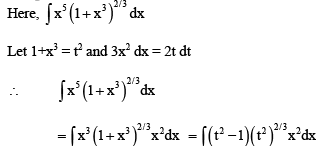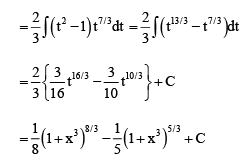Competition Level Test: Definite And Indefinite Integral - Question 24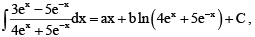then

Detailed Solution for Competition Level Test: Definite And Indefinite Integral - Question 24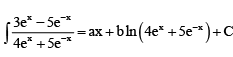Differentiating both sides, we get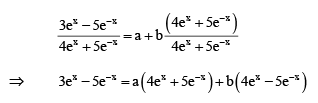Comparing the coefficient of like terms on both sides, we get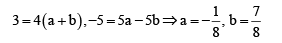Competition Level Test: Definite And Indefinite Integral - Question 25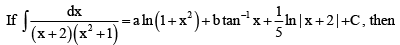Detailed Solution for Competition Level Test: Definite And Indefinite Integral - Question 25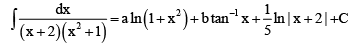Differentiating both sides, we get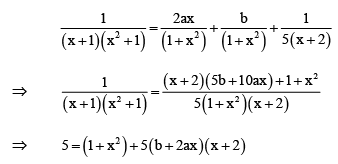Comparing the like powers of x in both sides, we get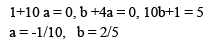Competition Level Test: Definite And Indefinite Integral - Question 26

If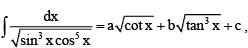then

Detailed Solution for Competition Level Test: Definite And Indefinite Integral - Question 26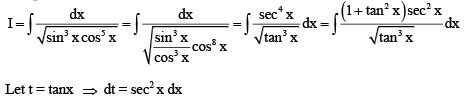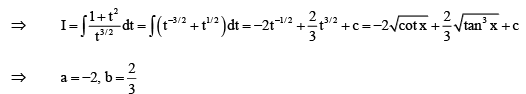Competition Level Test: Definite And Indefinite Integral - Question 27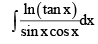is equal to

Detailed Solution for Competition Level Test: Definite And Indefinite Integral - Question 27

t = ln(tan x)
dt = (sec2 x)/(tan x) dx
=> (1/cos^2x) * (cosx /sinx) dx = dt
dt = dx/(cosx sinx)
I = ∫t dt
= [t2]/2 + c
= 1/2[ln(tanx)]2 + c

Competition Level Test: Definite And Indefinite Integral - Question 28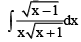is equal to

Detailed Solution for Competition Level Test: Definite And Indefinite Integral - Question 28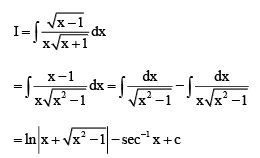Competition Level Test: Definite And Indefinite Integral - Question 29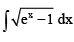is equal to

Detailed Solution for Competition Level Test: Definite And Indefinite Integral - Question 29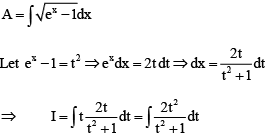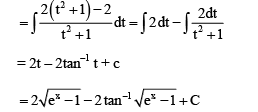Competition Level Test: Definite And Indefinite Integral - Question 30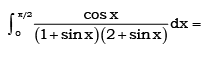Detailed Solution for Competition Level Test: Definite And Indefinite Integral - Question 30

ut sin x = t Þ cos x dx = dt, so that reduced integral is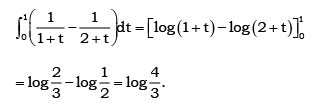## Mathematics (Maths) Class 12

209 videos|218 docs|139 tests
 Use Code STAYHOME200 and get INR 200 additional OFF Use Coupon Code
Information about Competition Level Test: Definite And Indefinite Integral Page
In this test you can find the Exam questions for Competition Level Test: Definite And Indefinite Integral solved & explained in the simplest way possible. Besides giving Questions and answers for Competition Level Test: Definite And Indefinite Integral, EduRev gives you an ample number of Online tests for practice

## Mathematics (Maths) Class 12

209 videos|218 docs|139 tests